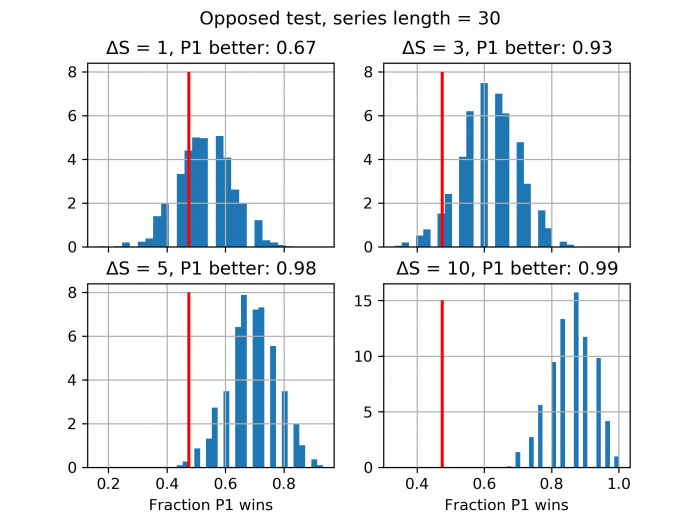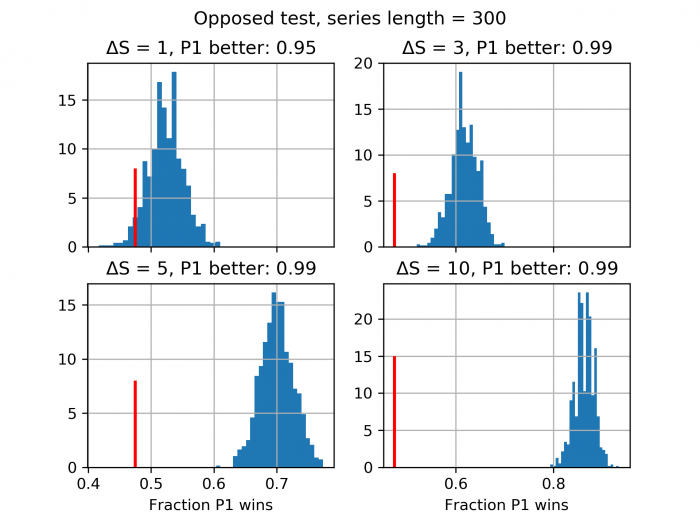# Dice math – What’s the difference?

Content warning: Sloppy statistics and a chance of horrible mistakes.

One interesting question regarding various mechanics is ‘How long does it take to figure out who is better?’ Characters in the world don’t observe each other’s bonuses but rather the results of the checks and checks have a random component in them. So it is very much possible that a more skilled character loses multiple times to a less skilled one. Luckily, we can use (or abuse) a few methods from statistics to take a stab at this problem.

P-values can be tricky- see replication crisis – but we are not looking to get rigorous answers here. It can be loosely defined as probability that the result we are seeing is due to a lucky choice of sample. So very sloppily: The lower the p-value, the more faith we can place in our hypothesis. In this case, our hypothesis is that one of the players has higher bonus than the other.

We do the following:

1. Generate a sample of N tests from a simple test d20 + $\Delta S$ – d20, and count the fraction of results P1 (i.e. the one with higher skill) wins.
2. Calculate p-value via Student’s t-test. The population mean we know from previous calculation for the case of equal skill.
3. repeat for different N and $\Delta S$

Then we want to test difference of means. If we call $\mu_0$ the mean number of victories for zero skill difference case and $\mu_1$ the mean number of victories for positive skill difference, our alternative hypothesis is $\mu_1 \neq \mu_0$.

Conventional choice for p-value limit is 0.05. This means (again, sloppily) that we are 95% confident that the result we are seeing is indeed real. Conversely, it means that 1 in 20 of results yielding p=0.05 is just due to chance. We’ll compare the results to this limit, but it shouldn’t be taken too seriously in this context (there are a couple of good example’s in wikipedia where you can get low p-values that don’t mean anything). So, if we see that p-values tend to be mostly low, it means we can distinguish the two contestants. If not, the result could be due to chance.

Now, this isn’t maximally rigorous and it’s been a while since I studied statistics/econometrics, but the results I see pass a certain sanity check. The behavior is what I’d expect, but see for yourself.

I want to stress that you shouldn’t place weight on individual points for smallish N, but rather look for a general trend. Let’s then get started. First, consider a simple opposed test as outlined above. If we see many large p-values, we should conclude that we might not be able to conclusively say who is better if we only know the results of N tests.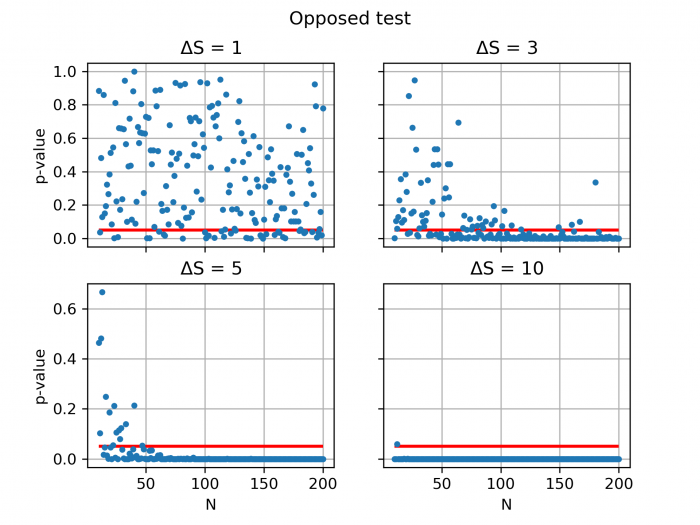As you can see, there is huge variance for low N (the red line signifies p=0.05). This is to be expected, since variance for small number of dice rolls is large. Consequence of this is that for small differences in skill, it can take 20-30 rolls until one character has decidedly better results than the other. On the other hand, for large differences, the more skilled contestant is apparent after very few tests.

There is also a different situation, where instead of opposed check, the player tests against a static target number. Let’s take a case of normalized target number of 12. Normalized here means that the lower bonus is deducted from the original target number so that only the skill difference matters. Performing similar analysis as before, we get the following.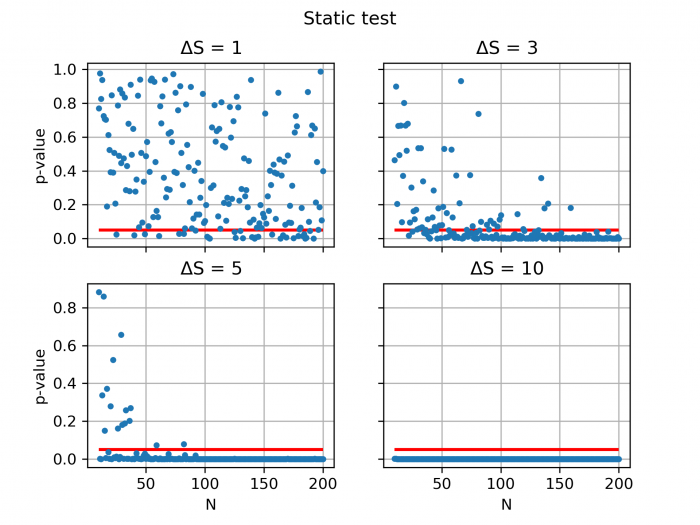So, again we get qualitatively similar results. For small numbers, it can take a long time – that is, many tests – to find out who is better. We can do the same for larger target number, say 15: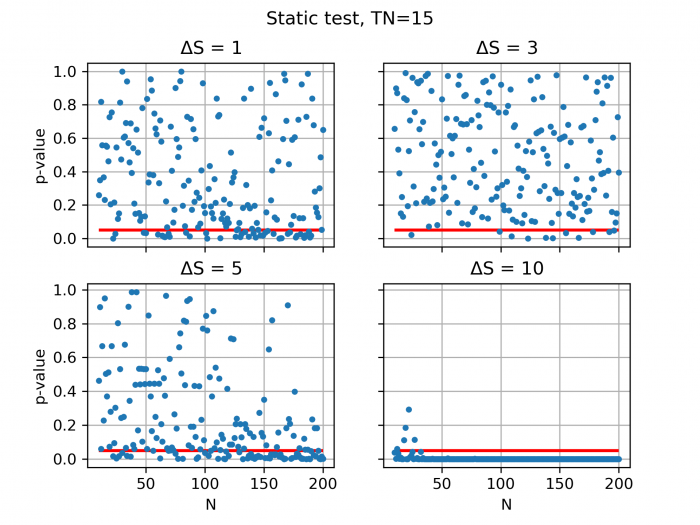Here we see something interesting. The target number is sufficiently high that getting a success is relatively rare for even the higher bonus, so it takes longer time for “the winner” to emerge convincingly.

The point of this exercise was to illustrate what does a difference in skill actually mean. We see that small differences in bonuses are not significant, especially for rare cases that might come up only a handful of times in a campaign. An example could be pure ability checks. Throughout first few levels, the difference in bonuses (in D&D or Pathfinder) is practically always less than 5. So it is very much possible that the mighty warrior performs worse than the scrawny wizard in strength tests throughout many sessions! This is doubly true for e.g. D&D5e, where numbers are generally small for skills and attack bonuses.

I want to stress again that this isn’t really rigorous and the individual points for small N can’t be trusted and there is also a nonzero chance that I have made a horrible mistake somewhere, but given that we know the underlying reality, the results are what we would expect.

Finally, here is an alternative way of looking at this. I’ve plotted the fraction of the tests P1 wins versus the length of the series.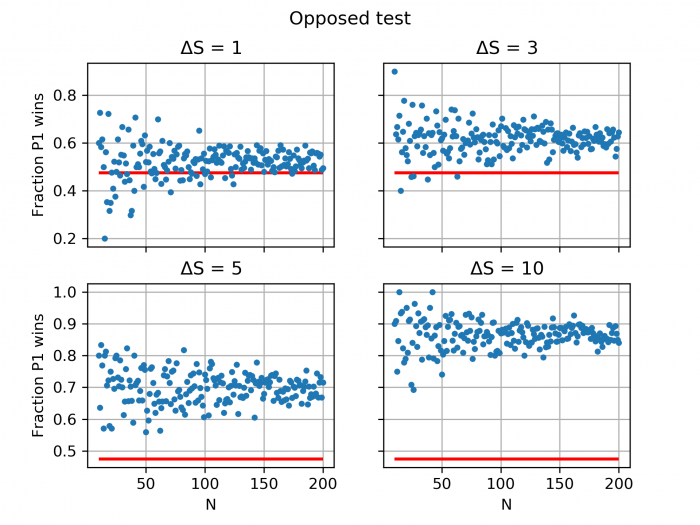The red line denotes the expected value for 0 skill difference. As you can see, for small difference, most of the values are close to the “zero difference” line, if not outright below it! So, if we impose the p<0.05 condition, we would expect many of the points for smaller skill bonuses to fail. So at the very least, on a casual look, this looks consistent with the earlier exploration.

The way to test how often larger skill wins using this methodology would be to generate a large number of length sets and fit a normal distribution that data and see what portion of that distribution falls above the 0 difference value. In principle, we don’t even need to do the fitting, and for now, we just do a simple comparison. The red line signifies 0-difference case and P1 better is the fraction of series, where P1 wins more often than 0-skill difference would indicate.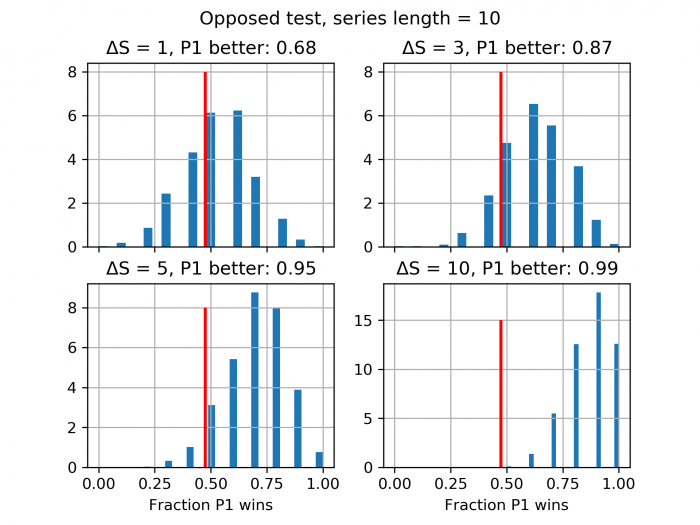I have also included a couple of more plots for different series lengths to demonstrate the effect. All in all, I’d say this is very consistent with what we saw using the sloppy p-value approach.